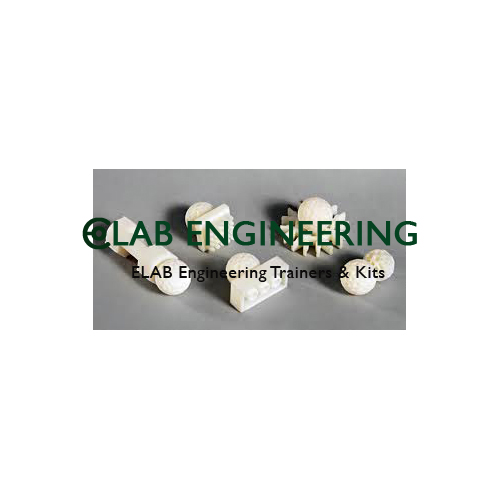# Geometrical Model Kit [Code: AME00169]Description & Specification:

Geometrical Model Kit.
Geometrical Model Kit a) Cube, (b) Cone, (c) Sphere, (d) Cylinder, (e) SquarePrism, (f) Triangular Prism (g) Square pyramid, (h)Octahedron, (I) Hexagonal Prism in two parts, 0)Tetrahedron, (k) pentagon, (I) Equilateral Triangle (m)Isosceles Triangle, (n) Right Angled Triangle, (0) Sphere, (p)Straight line. Set of Sixteen plastic models in a card board case.